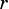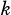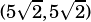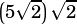Which of the following equations describes a circle with radius 10 that passes through the origin when graphed in the xy-plane?

A) (x – 5)² + (y+5)² = 10

B) (x – 5)² + (y+5)² = 100

C) (x – 10)² + (y+10)² = 10

D) (x – 5√2)² + (y+5√2)² = 100

Clearly, A) is out because that one does not have a radius of 10. What is the most time-efficient way to solve this? Sketch and eyeball?

The move here is to recognize that it’s a right triangle question. (There’s a subtle clue to this in the answer choices–see those‘s?)

You also need to know the basic circle equation,, whereis the circle’s center andis the radius, but from your question I sense that you have this bit down.

Recognize that when a circle goes through the origin, its radius will be the hypotenuse of a right triangle with legsand. Like so:So, which of the coordinates in the answer choices will result in a hypotenuse of 10? Only, which will have a hypotenuse of, which of course simplifies to 10.

Does that help?Robin says:Hsvwright says:infoanalysis says: